Examples

Chapter 10 Class 11 Straight Lines
Serial order wise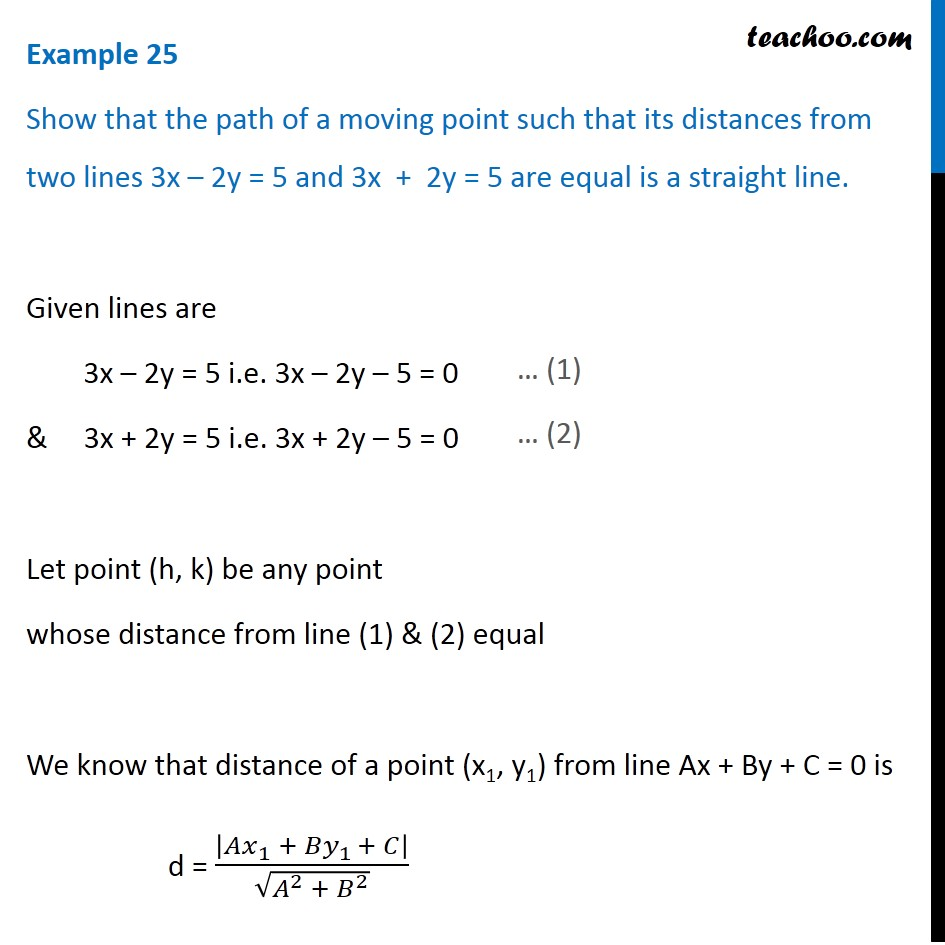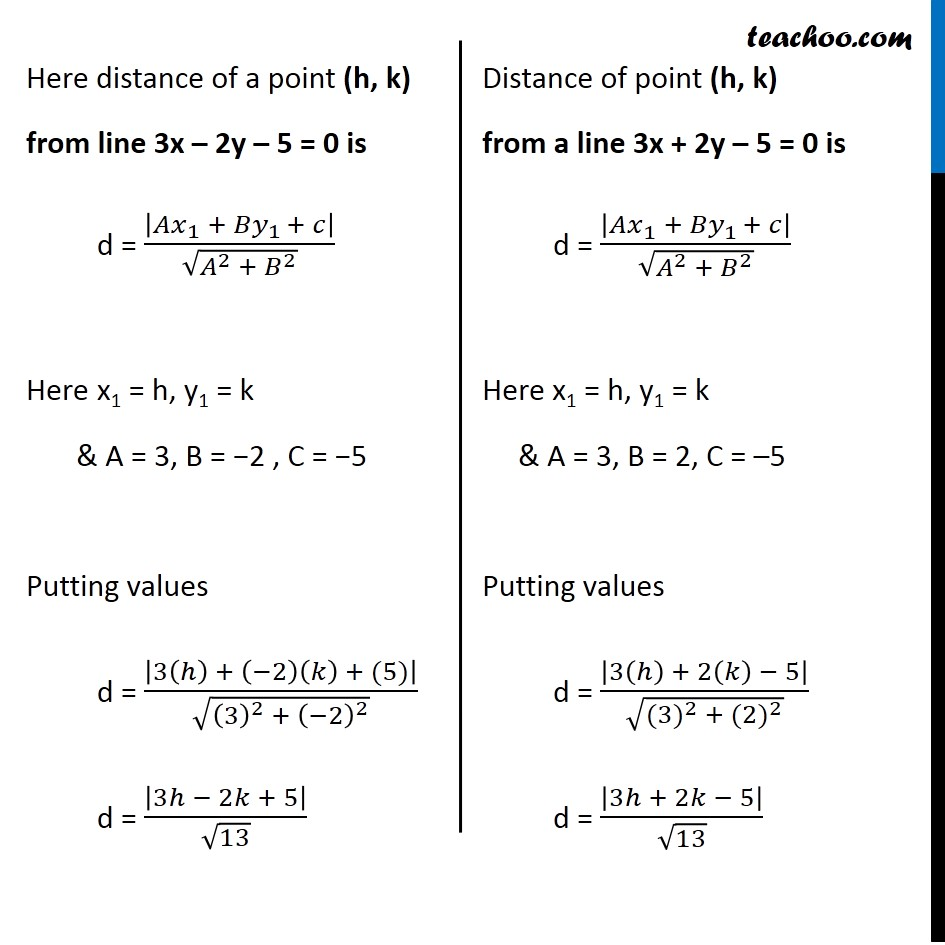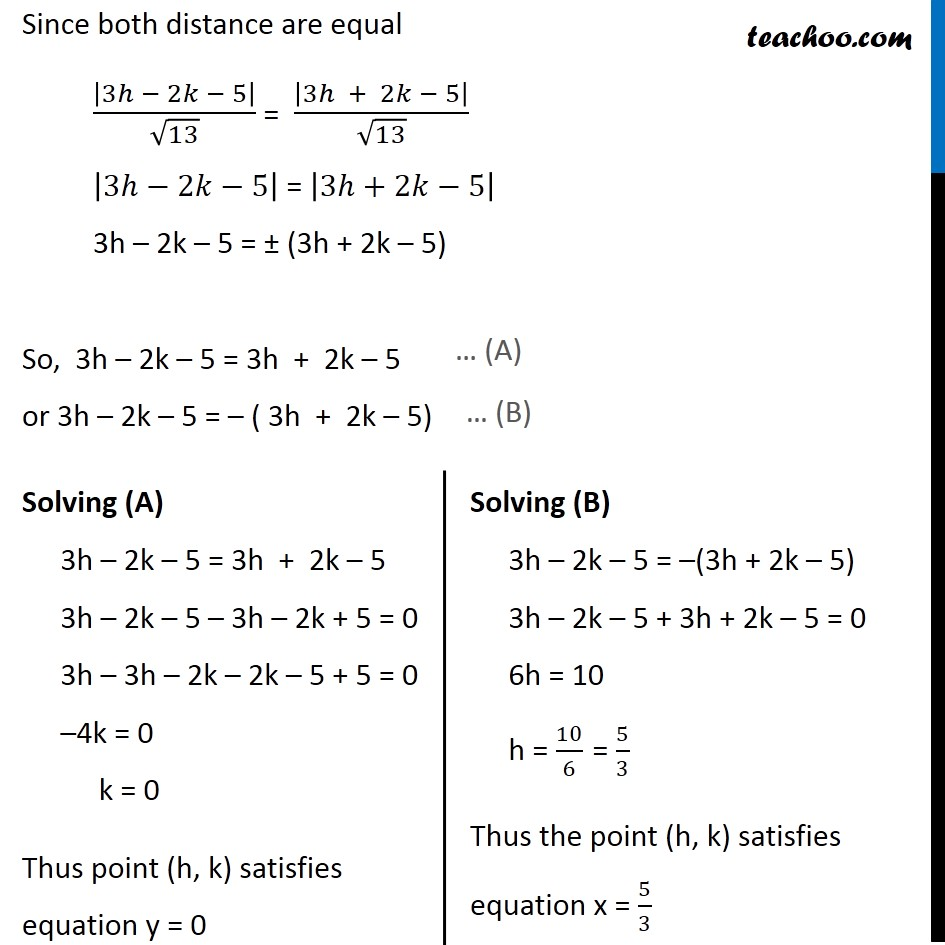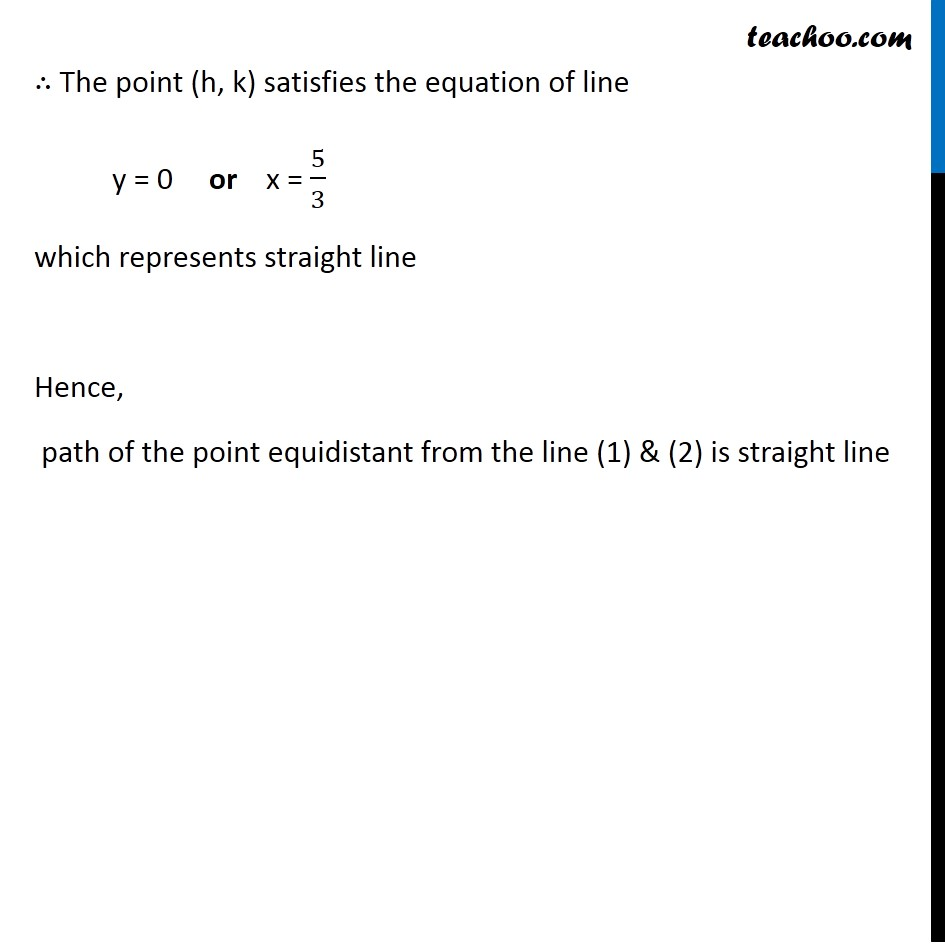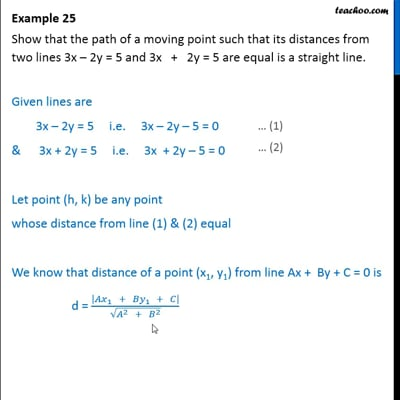This video is only available for Teachoo black users

Maths Crash Course - Live lectures + all videos + Real time Doubt solving!

### Transcript

Example 25 Show that the path of a moving point such that its distances from two lines 3x – 2y = 5 and 3x + 2y = 5 are equal is a straight line. Given lines are 3x – 2y = 5 i.e. 3x – 2y – 5 = 0 & 3x + 2y = 5 i.e. 3x + 2y – 5 = 0 Let point (h, k) be any point whose distance from line (1) & (2) equal We know that distance of a point (x1, y1) from line Ax + By + C = 0 is d = |〖𝐴𝑥〗_1 + 〖𝐵𝑦〗_1 + 𝐶|/√(𝐴^2 + 𝐵^2 ) Here distance of a point (h, k) from line 3x – 2y – 5 = 0 is d = |〖𝐴𝑥〗_1 + 〖𝐵𝑦〗_1 + 𝑐|/√(𝐴^2 + 𝐵^2 ) Here x1 = h, y1 = k & A = 3, B = −2 , C = −5 Putting values d = |3(ℎ) + (−2)(𝑘) + (5)|/√((3)^2 + (−2)^2 ) d = |3ℎ − 2𝑘 + 5|/√13 Distance of point (h, k) from a line 3x + 2y – 5 = 0 is d = |〖𝐴𝑥〗_1 + 〖𝐵𝑦〗_1 + 𝑐|/√(𝐴^2 + 𝐵^2 ) Here x1 = h, y1 = k & A = 3, B = 2, C = –5 Putting values d = |3(ℎ) + 2(𝑘) − 5|/√(〖(3)〗^2 + 〖(2)〗^2 ) d = |3ℎ + 2𝑘 − 5|/√13 Since both distance are equal |3ℎ − 2𝑘 − 5|/√13 = |3ℎ + 2𝑘 − 5|/√13 |3ℎ−2𝑘−5| = |3ℎ+2𝑘−5| 3h – 2k – 5 = ± (3h + 2k – 5) So, 3h – 2k – 5 = 3h + 2k – 5 or 3h – 2k – 5 = – ( 3h + 2k – 5) Solving (A) 3h – 2k – 5 = 3h + 2k – 5 3h – 2k – 5 – 3h – 2k + 5 = 0 3h – 3h – 2k – 2k – 5 + 5 = 0 –4k = 0 k = 0 Thus point (h, k) satisfies equation y = 0 Solving (B) 3h – 2k – 5 = –(3h + 2k – 5) 3h – 2k – 5 + 3h + 2k – 5 = 0 6h = 10 h = 10/6 = 5/3 Thus the point (h, k) satisfies equation x = 5/3 ∴ The point (h, k) satisfies the equation of line y = 0 or x = 5/3 which represents straight line Hence, path of the point equidistant from the line (1) & (2) is straight line